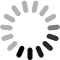Understanding Inertia Ratio in Synchronous Motor Control

When sizing a motor for a particular application, the general rule of thumb is to keep the inertia ratio below 10:1—meaning the motor moves a load 10X heavier than its rotor. If the ratio is too high, one solution is to add a gearbox to reduce inertia. However, this increases the amount of energy used. This paper presents a detailed quantitative comparison of response times between low-inertia and medium-inertia motors in both rigid and elastic systems, with calculations of limit criteria for inertia ratios. How to measure the energy consumption of gearboxes is explained, showing why choosing the right gearbox can achieve significant savings.

Reference

:

Date

:

Type

:

Languages :

Version :